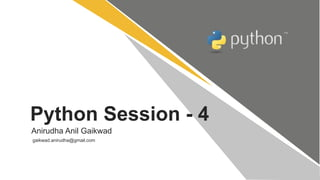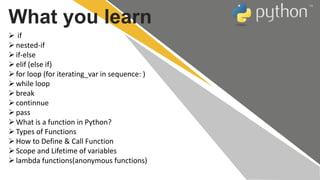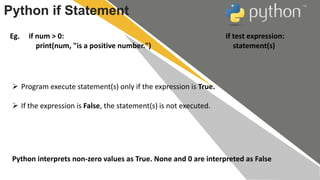O slideshow foi denunciado.
Seu SlideShare está sendo baixado. ×

# Python Session - 4Carregando em…3
×

1 de 27 Anúncio
1 de 27 Anúncio

# Python Session - 4

Python Session - 4
if
nested-if
if-else
elif (else if)
for loop (for iterating_var in sequence: )
while loop
break
continnue
pass
What is a function in Python?
Types of Functions
How to Define & Call Function
Scope and Lifetime of variables
lambda functions(anonymous functions)

Python Session - 4
if
nested-if
if-else
elif (else if)
for loop (for iterating_var in sequence: )
while loop
break
continnue
pass
What is a function in Python?
Types of Functions
How to Define & Call Function
Scope and Lifetime of variables
lambda functions(anonymous functions)

### Python Session - 4

1. 1. Python Session - 4 Anirudha Anil Gaikwad gaikwad.anirudha@gmail.com
2. 2.  if nested-if if-else elif (else if) for loop (for iterating_var in sequence: ) while loop break continnue pass What is a function in Python? Types of Functions How to Define & Call Function Scope and Lifetime of variables lambda functions(anonymous functions) What you learn
3. 3. Python if Statement Eg. if num > 0: print(num, "is a positive number.") if test expression: statement(s)  Program execute statement(s) only if the expression is True.  If the expression is False, the statement(s) is not executed. Python interprets non-zero values as True. None and 0 are interpreted as False
4. 4. Python Nested if statements  Nested if statements enable us to use if ? else statement inside an outer if statement.  Any number of if statements can be nested inside one another. Indentation is the only way to figure out the level of nesting. num = float(input("Enter a number: ")) if num >= 0: if num == 0: print("Zero") else: print("Positive number") else: print("Negative number")
5. 5. Python if...else Statement if test expression: Body of if else: Body of else if num >= 0: print("Positive or Zero") else: print("Negative number")  The if..else statement evaluates expression and will execute body of if only when test condition is True.  If the condition is False, body of else is executed. Indentation is used to separate the blocks.
6. 6. Python if...elif...else Statement if test expression: Body of if elif test expression: Body of elif else: Body of else if num > 0: print("Positive number") elif num == 0: print("Zero") else: print("Negative number")  The elif is short for else if. It allows us to check for multiple expressions.  If the condition for if is False, it checks the condition of the next elif block and so on.  If all the conditions are False, body of else is executed.  The if block can have only one else block. But it can have multiple elif blocks
7. 7.  The for loop in Python is used to iterate over a sequence (list, tuple, string) or other iterable objects. Iterating over a sequence is called traversal. for loop in Python for iterating_var in sequence: statement(s)  Loop continues until we reach the last item in the sequence. The body of for loop is separated from the rest of the code using indentation. i=1 n=int(input("Enter the number up to which you want to print :")) for i in range(0,10): print(i,end = ' ')
8. 8. while loop in Python  The while loop in Python is used to iterate over a block of code as long as the test expression (condition) is true. while test_expression: Body of while  In while loop, test expression is checked first. The body of the loop is entered only if the test_expression evaluates to True. After one iteration, the test expression is checked again. This process continues until the test_expression evaluates to False. while i <= n: sum = sum + i i = i+1 # update counter print("The sum is", sum) i=1; while i<=10: print(i); i=i+1;
9. 9. Python break statement  The break statement terminates the loop containing it. Control of the program flows to the statement immediately after the body of the loop.  If break statement is inside a nested loop (loop inside another loop), break will terminate the innermost loop. list =[1,2,3,4] count = 1; for i in list: if i == 4: print("item matched") count = count + 1; break print("found at",count,"location");
10. 10. Python continue statement  The continue statement is used to skip the rest of the code inside a loop for the current iteration only. Loop does not terminate but continues on with the next iteration. i=1; #initializing a local variable for i in range(1,11): if i==5: continue; print("%d"%i);
11. 11. pass statement in Python  The pass statement is a null operation since nothing happens when it is executed. It is used in the cases where a statement is syntactically needed but we don't want to use any executable statement at its place.  For example, it can be used while overriding a parent class method in the subclass but don't want to give its specific implementation in the subclass.  Pass is also used where the code will be written somewhere but not yet written in the program file. list = [1,2,3,4,5] flag = 0 for i in list: print("Current element:",i,end=" "); if i==3: pass; print("nWe are inside pass blockn"); flag = 1; if flag==1: print("nCame out of passn"); flag=0;
12. 12. What is a function in Python? In Python, function is a group of related statements that perform a specific task. Functions help break our program into smaller and modular chunks. As our program grows larger and larger, functions make it more organized and manageable. Furthermore, it avoids repetition and makes code reusable. def function_name(parameters): """docstring""" statement(s)
13. 13.  Keyword def marks the start of function header.  A function name to uniquely identify it. Function naming follows the same rules of writing identifiers in Python.  Parameters (arguments) through which we pass values to a function. They are optional.  A colon (:) to mark the end of function header.  Optional documentation string (docstring) to describe what the function does.  One or more valid python statements that make up the function body. Statements must have same indentation level (usually 4 spaces).  An optional return statement to return a value from the function. Function definition consists of following components. What is a function in Python?
14. 14. How to call a function in python? Once we have defined a function, we can call it from another function, program or even the Python prompt. To call a function we simply type the function name with appropriate parameters.
15. 15.  Functions that we define ourselves to do certain specific task are referred as user-defined functions.  Functions that readily come with Python are called built-in functions. # Program to illustrate # the use of user-defined functions def add_numbers(x,y): sum = x + y return sum num1 = 5 num2 = 6 print("The sum is", add_numbers(num1, num2)) Types of function in python?
16. 16. Most of the programming languages like C, C++, Java use braces { } to define a block of code. Python uses indentation. A code block (body of a function, loop etc.) starts with indentation and ends with the first unindented line. The amount of indentation is up to you, but it must be consistent throughout that block. Generally four whitespaces are used for indentation and is preferred over tabs. Here is an example. Python Indentation for i in range(0,10): print(i) if i == 5: break
17. 17. Scope and Lifetime of variables Scope of a variable is the portion of a program where the variable is recognized. Parameters and variables defined inside a function is not visible from outside. Hence, they have a local scope.  Lifetime of a variable is the period throughout which the variable exits in the memory. The lifetime of variables inside a function is as long as the function executes.  The variable defined outside any function is known to have a global scope variable. x = "global variable" def foo(): global x #global variable access in function y = "local variable" x = x * 2 print(x) print(y) foo()
18. 18. Scope and Lifetime of variables The basic rules for global keyword in Python are:  When we create a variable inside a function, it’s local by default.  When we define a variable outside of a function, it’s global by default. You don’t have to use global keyword.  We use global keyword to read and write a global variable inside a function.  Use of global keyword outside a function has no effect
19. 19.  Nonlocal Variables Nonlocal variable are used in nested function whose local scope is not defined. This means, the variable can be neither in the local nor the global scope. We use nonlocal keyword to create nonlocal variable. Scope and Lifetime of variables def outer(): x = "local" def inner(): nonlocal x x = "nonlocal" print("inner:", x) inner() print("outer:", x) outer() #If we change value of nonlocal variable, the changes appears in the local variable
20. 20. What is recursion in Python? Recursion is the process of defining something in terms of itself. Python Recursive Function : We know that in Python, a function can call other functions. It is even possible for the function to call itself. These type of construct are termed as recursive functions. # An example of a recursive function to # find the factorial of a number def calc_factorial(x): """This is a recursive function to find the factorial of an integer""" if x == 1: return 1 else: return (x * calc_factorial(x-1)) num = 4 print("The factorial of", num, "is", calc_factorial(num))
21. 21.  Advantages of Recursion Recursive functions make the code look clean and elegant. A complex task can be broken down into simpler sub-problems using recursion. Sequence generation is easier with recursion than using some nested iteration.  Limitations of Recursion Sometimes the logic behind recursion is hard to follow through. Recursive calls are expensive (inefficient) as they take up a lot of memory and time. Recursive functions are hard to debug. What is recursion in Python?
22. 22. lambda functions(anonymous functions) The anonymous functions are declared by using lambda keyword. However, Lambda functions can accept any number of arguments, but they can return only one value in the form of expression. lambda arguments: expression # Program to show the use of lambda functions double = lambda x: x * 2 # Output: 10 print(double(5))
23. 23. Use of Lambda Function in python We use lambda functions when we require a nameless function for a short period of time. In Python, we generally use it as an argument to a higher-order function (a function that takes in other functions as arguments). Lambda functions are used along with built-in functions like filter(), map() etc. lambda functions(anonymous functions)
24. 24.  Example use with filter() The filter() function in Python takes in a function and a list as arguments. The function is called with all the items in the list and a new list is returned which contains items for which the function evaluats to True. Here is an example use of filter() function to filter out only even numbers from a list. # Program to filter out only the even items from a list my_list = [1, 5, 4, 6, 8, 11, 3, 12] new_list = list(filter(lambda x: (x%2 == 0) , my_list)) # Output: [4, 6, 8, 12] print(new_list) lambda functions(anonymous functions)
25. 25. Example use with map() The map() function in Python takes in a function and a list. The function is called with all the items in the list and a new list is returned which contains items returned by that function for each item. Here is an example use of map() function to double all the items in a list. lambda functions(anonymous functions) # Program to double each item in a list using map() my_list = [1, 5, 4, 6, 8, 11, 3, 12] new_list = list(map(lambda x: x * 2 , my_list)) # Output: [2, 10, 8, 12, 16, 22, 6, 24] print(new_list)
26. 26. T H A N K S